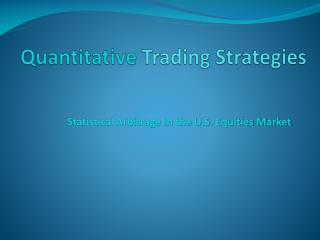# Quantitative Trading Strategies - PowerPoint PPT PresentationDownload PresentationDownload Presentation- - - - - - - - - - - - - - - - - - - - - - - - - - - E N D - - - - - - - - - - - - - - - - - - - - - - - - - - -
##### Presentation Transcript

1. Quantitative Trading Strategies Statistical Arbitrage in the U.S. Equities Market

2. ETF approach: Using industries • A diverse set of ETFs can be used to explain the returns of a stock • Multiple regression model will be of the form:

3. The PCA approach • Principle Component Analysis to extract factors from the return data • Eigenvectors are the principle components of the data and its corresponding eigenvalues are its variance. • Each principle components are a linear combination of all the stocks in the data and can be regarded as an eigenportfolio • The returns of each eigenportfolio can be used in the multiple regression analysis

4. Differences between PCA and ETF approaches • ETF approach requires some prior understanding of the economical situation to know the “appropriate” set of ETFs needed • PCA factor loadings is more intuitive than for ETF • ETF gives more weight to large capitalization companies, whereas PCA has no a priori capitalization bias

5. Trading Signals • Using the OU parameters, we can obtain the mean and variance of X and define the dimensionless variable: • Above equation is known as the s-score which measures the distance to equilibrium of the co-integrated residual in units of standard deviations. The signals are as follow: • buy to open if si < −sbo • sell to open if si> +sso • close short position if si < +sbc • close long position si > −ssc

6. Results from using PCA approach • The stock MCK gives the best result. The trading strategy performs well throughout the 3-year period with a profit of almost \$754,000 at the end of the trading period. • The optimized trading levels sbo, sbo, sbc, sscobtained are 1, 0.25, 1, 0.25 respectively.

7. Results from using PCA approach • Second best performing stock is “IP” with an Omega of 1.33. The P&L over the three year period is shown below. • A profit of almost \$811,000 at the end of the trading period. The optimized trading levels are same as before.

8. Results from using ETF approach • Among the 100 stocks, NRG gives the best results. The trading strategy performs well throughout the 3-year period with a profit of almost \$1,730,000 at the end of the trading period. • The optimized trading levels sbo, sbo, sbc, sscobtained are 1, 0.25, 1, 0.25 respectively.

9. Results from using ETF approach • Second best performing stock is “ATI” with an Omega of 1.47. The P&L over the three year period is shown below. • A profit of almost \$1,590,000 at the end of the trading period. The optimized trading levels are same as before. • Video of P&L using ETF Approach

10. Backtest: Bootstrapping

11. Backtest: Bootstrapping

12. Backtest: Outsample Test

13. New Findings • We find that the optimized trading levels sbo, sbo, sbc, sscobtained are 1, 0.25, 1, 0.25 respectively as opposed to what is being proposed in the paper • For the optimized set of parameters we got very good profit in both the approaches (ranging above 80%) • Omega achieved from out-sample testing was not good enough to support the strategy • This is further cemented by the results from bootstrapping • Please run ‘main_ETF.m’ to see the results.